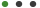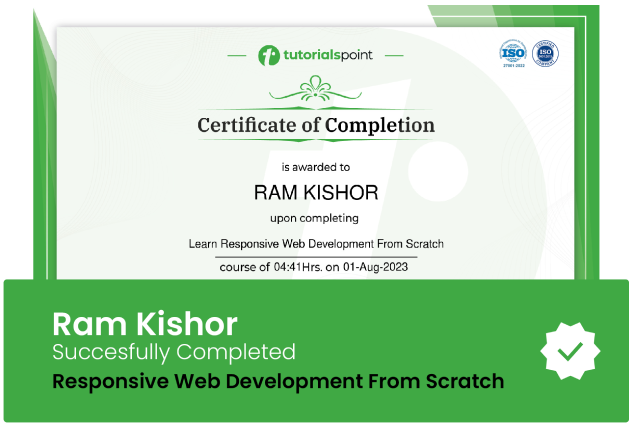Ahmed Mahdy

Learn basics of MATLAB Simulink to simulate different electric components in MATLAB Simulink for electrical engineeringUpdated on Sep, 2023Language - EnglishAhmed MahdyTeaching & Academics,MATLAB30-days Money-Back Guarantee

Training 5 or more people ?

## Course Description

The only course out there with everything you need to know about MATLAB Simulink from A to Z.

Throughout the course, you will the simulation using MATLAB of:

• Single-phase half wave controlled rectifier.

• Single-phase bridge controlled rectifier.

• Single-phase AC chopper with R and RL load.

• A DC-DC converter as buck regulator.

• Boost regulator.

• Buck-Boost regulator.

• Single-phase half-bridge inverter.

• Single-phase bridge inverter.

• Three Phase Inverter.

• PV cell in solar energy using Simulink tool in MATLAB.

• How to obtain a complete grid-connected PV system in MATLAB.

• You will learn about separately excited DC Machines and how to:

• Model the DC machine in a no-load case using Simulink in MATLAB.

• Model the DC machine in presence of load torque using Simulink in MATLAB.

• Simulating the DC machine using the power library from Simulink in MATLAB.

• You will learn about Induction motors as:

• Construction and principle of operation of induction motor.

• Torque-speed characteristics of induction motor.

• Equivalent circuit and power flow of induction motor.

• Simulation of induction motor using Simulink in MATLAB.

• MATLAB simulation of the wind turbine.

• Cp plotting and lookup table in MATLAB.

If you've been looking for ONE COURSE with in-depth insight into MATLAB Simulation, take this course.

### Goals

What will you learn in this course:

• Learn simulation of power electronics circuits using Simulink in MATLAB

• Understand how to simulate AC-DC converters as half and full bridge rectifiers using Simulink in MATLAB

• Understand how to simulate AC-AC converters as single phase AC choppers using Simulink in MATLAB

• Learn how to simulate DC-DC converters as buck, boost and buck-boost regulators using Simulink in MATLAB

• Simulate DC-AC converters such as half, full bridge and three phase inverters using Simulink in MATLAB

• Learn how to simulate PV cell using Simulink in MATLAB

• Learn about separately excited DC machine and how to simulate it using Simscape and how to model it using Simulink in MATLAB

• Learn about different converters principle of operation such AC choppers, DC choppers, inverters and rectifiers

• MATLAB simulation of the wind turbine

• Cp plotting and lookup table in MATLAB

• How to obtain a complete grid-connected PV system in MATLAB

### Prerequisites

What are the prerequisites for this course?

• You should have a basic mathematical background## Curriculum

Check out the detailed breakdown of what’s inside the course

Power Electronics Simulations Using Simulink In MATLAB
23 Lectures
•Introduction to MATLAB Simulations Using Simulink 03:38 03:38
•Half Wave Controlled Rectifier Principle of Operation 04:42 04:42
•Simulation of Half Wave Controlled Rectifier In MATLAB 25:33 25:33
•Bridge Controlled Rectifier Principle of Operation Part 1 06:01 06:01
•Bridge Controlled Rectifier Principle of Operation Part 2 05:47 05:47
•Simulation of Bridge Controlled Rectifier in MATLAB 16:18 16:18
•AC Chopper with R Load Principle of Operation 14:13 14:13
•Simulation of AC Chopper with R and RL Loads in MATLAB 10:46 10:46
•Buck Regulator Principle Of Operation Part 1 16:18 16:18
•Buck Regulator Principle of Operation Part 2 16:46 16:46
•Simulation of Buck Regulator in MATLAB 14:05 14:05
•Boost Regulator Principle of Operation 23:06 23:06
•Simulation of Boost Regulator in MATLAB 12:11 12:11
•Buck-Boost Regulator Principle of Operation 17:27 17:27
•Simulation of Buck-Boost Regulator in MATLAB 09:23 09:23
•Single Phase Half Bridge Principle of Operation 15:28 15:28
•Simulation of Single Phase Half Bridge Inverter in MATLAB 17:30 17:30
•Single Phase Bridge Principle of Operation 05:40 05:40
•Simulation of Single Phase Bridge Inverter in MATLAB 09:53 09:53
•Three Phase Inverter Obtaining the Line Voltage 14:50 14:50
•Three Phase Inverter Obtaining the Phase Voltage 16:57 16:57
•Simulation of Three Phase Inverter in MATLAB 17:03 17:03
•Simulation of Charging and Discharging Capacitor Using MATLAB 10:25 10:25
Solar Energy Simulation Using Simulink In MATLAB
2 LecturesWind Energy Simulation Using Simulink In MATLAB
8 LecturesDC Motor Simulation Using Simulink In MATLAB
4 LecturesInduction Motor Simulation Using Simulink In MATLAB
4 LecturesSynchronous Generator Simulation In Simulink Of MATLAB
4 LecturesPower System Simulations
1 LecturesPID Controller In MATLAB
2 Lectures## Instructor DetailsAhmed Mahdy

Electrical Power Engineer

I am Ahmed Mahdy an electrical power engineer, I am also an online instructor at more than 20 platforms.

I am also the founder of the "Khadija Academy" website for electrical engineering courses.

I have a YouTube educational engineering channel called" Khadija Academy", I regularly post videos related to electrical engineering.

I am also an author on Amazon Kindle having 6 books about electrical engineering.

In 2018 Udemy awarded me of being from the top 10% most engaging instructors.

I also created Khadija Academy  in 2019, my mission is "Learn the fundamentals of electrical engineering branches from absolute beginner to advanced level."

In every one of my Udemy courses, you'll see that I am always available and always offer you support through each course. I will join your success and help you through any obstacles.

You'll notice that my courses get many 5-star ratings & reviews and my student satisfaction ratings also reflect this.

In the many positive course reviews, my courses get, most people like the Way I explain everything and the encouraging teaching style that I convey as well as how I get right to the point and walk you, step-by-step through Complex Ideas.

(You'll find my courses below this bio - and all of my courses have at least 10 minutes of free previews available to you, so you can quickly determine the courses that are right for you)

My courses can be watched 24/7 wherever you are.

You can also view them on mobile devices with the Udemy mobile app.

## Course Certificate

User your certification to make a career change or to advance in your current career. Salaries are among the highest in the world.